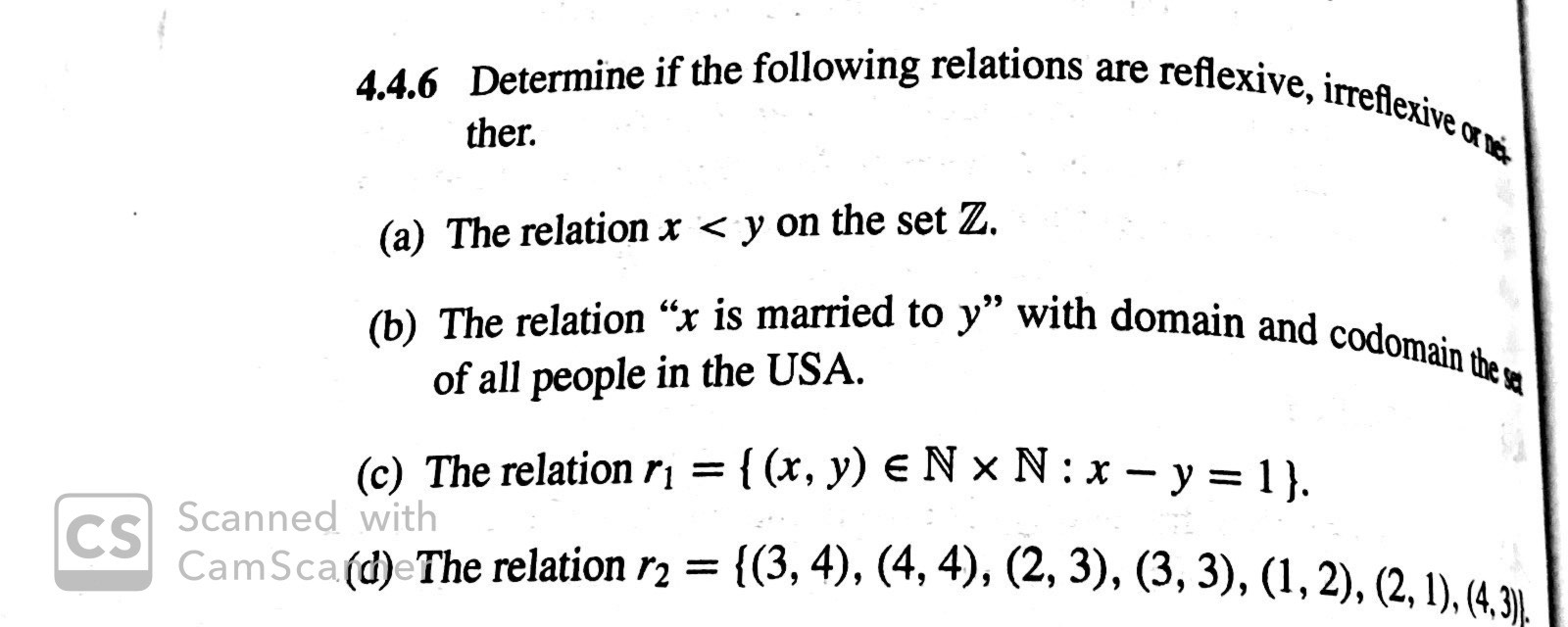# 4.4.6 Determine if the following relations are reflexive, irreflexive other(a) The relation x < y on the set Z.(b) The relation "x is married to y" with domain and codomain the aof all people in the USA(c) The relation r= { (x, y) e N x N: x-y = 1 }.Scanned withCSCamSca (d)eThe relation r2 ={(3, 4), (4,4), (2, 3), (3, 3), (1,2), (2,1), (4,3).

Question
14 viewshelp_outlineImage Transcriptionclose4.4.6 Determine if the following relations are reflexive, irreflexive o ther (a) The relation x < y on the set Z. (b) The relation "x is married to y" with domain and codomain the a of all people in the USA (c) The relation r= { (x, y) e N x N: x-y = 1 }. Scanned with CS CamSca (d)eThe relation r2 = {(3, 4), (4,4), (2, 3), (3, 3), (1,2), (2,1), (4,3). fullscreen
check_circle

Step 1

According to the given information it is required to check whether the relation is reflexive, irreflexive or neither.

A binary relation R over the set X is reflexive if every element of X is related to itself.

And

A binary relation R over the set X is irreflexive if it does not relate any element to itself.

For part (a)

The relation x < y on the set Z

There is no element belong to Z such that x < x so, the relation is irreflexive as no element relate to itself.

Step 2

For part (b)

The relation x is married to y with domain and codomain the set of all people in USA

It is not possible that any person can marry to itself so, the relation is irreflexive as no element relate to itself.

Step 3

For part (...

### Want to see the full answer?

See Solution

#### Want to see this answer and more?

Solutions are written by subject experts who are available 24/7. Questions are typically answered within 1 hour.*

See Solution
*Response times may vary by subject and question.
Tagged in

### Math